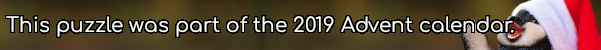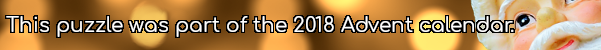mscroggs.co.uk
mscroggs.co.uksubscribe

# Puzzles

## 22 DecemberIn bases 3 to 9, the number 112 is: $$11011_3$$, $$1300_4$$, $$422_5$$, $$304_6$$, $$220_7$$, $$160_8$$, and $$134_9$$. In bases 3, 4, 6, 8 and 9, these representations contain no digit 2.
There are two 3-digit numbers that contain no 2 in their representations in all the bases between 3 and 9 (inclusive). Today's number is the smaller of these two numbers.

## 22 DecemberIn base 2, 1/24 is 0.0000101010101010101010101010...
In base 3, 1/24 is 0.0010101010101010101010101010...
In base 4, 1/24 is 0.0022222222222222222222222222...
In base 5, 1/24 is 0.0101010101010101010101010101...
In base 6, 1/24 is 0.013.
Therefore base 6 is the lowest base in which 1/24 has a finite number of digits.
Today's number is the smallest base in which 1/10890 has a finite number of digits.
Note: 1/24 always represents 1 divided by twenty-four (ie the 24 is written in decimal).

## 121

Find a number base other than 10 in which 121 is a perfect square.
Tags: numbers, bases

Let $$a_b$$ denote $$a$$ in base $$b$$.
Find bases $$A$$, $$B$$ and $$C$$ less than 10 such that $$12_A+34_B=56_C$$.
Tags: numbers, bases

## Reverse bases again

Find three digits $$a$$, $$b$$ and $$c$$ such that $$abc$$ in base 10 is equal to $$cba$$ in base 9?
Tags: numbers, bases

## Reverse bases

Find two digits $$a$$ and $$b$$ such that $$ab$$ in base 10 is equal to $$ba$$ in base 4.
Find two digits $$c$$ and $$d$$ such that $$cd$$ in base 10 is equal to $$dc$$ in base 7.
Find two digits $$e$$ and $$f$$ such that $$ef$$ in base 9 is equal to $$fe$$ in base 5.
Tags: numbers, bases

## Archive

Show me a random puzzle
▼ show ▼